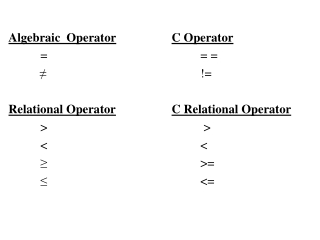DownloadDownload PresentationAlgebraic Operator C Operator = = = ≠ !=

# Algebraic Operator C Operator = = = ≠ !=

Télécharger la présentation## Algebraic Operator C Operator = = = ≠ !=

- - - - - - - - - - - - - - - - - - - - - - - - - - - E N D - - - - - - - - - - - - - - - - - - - - - - - - - - -
##### Presentation Transcript

1. Algebraic OperatorC Operator = = = ≠ != Relational OperatorC Relational Operator > > < < ≥ >= ≤ <=

2. Datatypes DatatypeKeywordBytes integer short 1 int 2 long 4 float float 4 double 8

3. Type Casting The operator called a cast operator is used to convert from one data type to another. Example: int a = 15; int b = 16; float c = 0.0; c = ( float ) a / b ; The calculation a / b produces an integer result which is then converted to a float for an accurate solution.

4. % f • %f used to represent floats without specifying precision. • Format: % a.b f b specifies the number of places after the decimal point (after rounding the result) a specifies the total field width (places before + after the decimal point)

5. Selection structures A selection structure is used to choose among alternative courses of action. The if selection structure executes an indicated action only when the condition is true. The if selection structure expects only one statement in its body. To include several statements, enclose the set of statements in { }. Compound statement - The set of statements within { }

6. Format: if (expression) statement; A decision is made based on the expression. If the expression evaluates to zero, it is treated as false. If the expression evaluates to nonzero, it is treated as true.

7. Format: if (expression) { statement1; statement2; statementn; }

8. Example: if ( a = = b ) printf(“The two numbers are equal.\n”); else printf(“The two numbers are different.\n”);

9. Nested if/else structures test for different cases. Example: if ( grade >= 90) printf(“ A \n”); else if ( grade >= 80) printf(“ B \n”); else if ( grade >= 70) printf(“ C \n”); else if ( grade >= 60) printf(“ D \n”); else printf(“F \n”);

10. The while Repetition Structure • A Repetition Structure allows the programmer to specify that an action is to be repeated while some condition remains true. • Format : while ( expression ) statement; • The while structure body may be a single statement or a compound statement. • The expression is tested each time through the loop. The statement is repeated until the expression remains true.

11. When the condition becomes false, the while condition is exited and control passes to the next statement in the program. • Example: Sum of the first 5 numbers: j = 1; sum = 0; while ( j <= 5) { sum = sum + j; j = j + 1; } printf(“sum is %d \n”, sum);

12. Increment and Decrement Operators • Unary increment operator ++ • Unary decrement operator -- • These operators are used when a variable is incremented or decremented by 1. OperatorExample ++ ++a ++ a++ -- --b -- b--

13. Conditional Operator • Conditional operator ?: • The conditional operator takes three operands (ternary operator). • Format: condition ? Value if true : Value if false; • Example: choice = number != 3 ? 0 : 3;